# Macintosh IFS manual

Written by Paul Bourke
May 1990

Introduction

The following describes iterated function systems, a particular implementation on the Macintosh, and presents some examples.

One of the many ways of describing the affine transformations for iterated functions systems (IFS) is as follows:

x = r cos(theta) x + s sin(phi) y + h
y = -r sin(theta) x + s cos(phi) y + k

Generally there are a number of these mappings (different values of r, s, theta, phi, h, k) which are chosen at random at each iteration.

How does the program work

The fundamental iterative process involves replacing rectangles with a series of rectangles called the generator. The rectangles are replaced by a suitably scaled, translated, and rotated version of the generator.

For example consider the generator belowIt consists of three rectangles, each with its own center, dimensions and rotation angle. The initial conditions usually consist of a single square, the first iteration then consists of replacing this square by a suitably positioned, scaled and rotated version of the above generator. The result would just be the generator above. The next iteration involves replacing each of the rectangles in the current system by suitabled positioned, scaled, and rotated versions of the generator resulting in the followingThe next iteration replaces each rectangle above again by the initial generator as shown below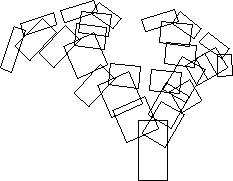and so on, three iterations laterIFS allows the user to explore the result of applying different generators. The generator rectangles can be created, shifted, scaled, and rotated by the handles on each rectangle shown below.The user may iterate forward or in reverse, colour the borders, interior, or background.

A library of interesting generators is provided for initial experimentation. Generators created by users may be saved in files and recalled at a later time.

Images so created may be copied from IFS and pasted into a more standard drawing package for incorporation into a document and printing.

Hopalong or "The Chaos Game"

A technique exists by which the resulting form after an infinite number of iterations can be derived. This is a function of the form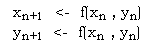This gives a series of (x,y) points all which lie on the result of an infinite IFS. Although it still takes an infinite number of terms in this series to form the result the appearance can be readily appreciated after a modest number of terms (10000 say).Note that with both methods it is possible to create the image at any scale. In many but not all cases zoomed in examples will be exhibit self similarity at all scales.

Applications

Data reduction for model files. If a generator can be found for a complex image then storing the generator and the rules of production results in a great deal of data reduction. For example the weed in the examples above might eventually contain over 2000 rectangles but is completely specified by the characteristics of 3 rectangles, only 5 numbers, center (cx,cy), scale (sx,sy), and angle

Note: it is not necessarily trivial to derive a rectangular generator for an arbitrary form, although it is possible to create a polygonal generator for any form. The following shows a maple leaf generated using the IFS program.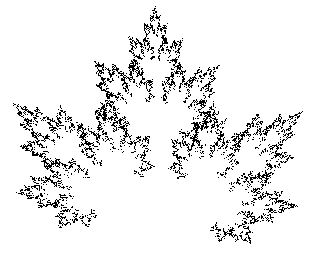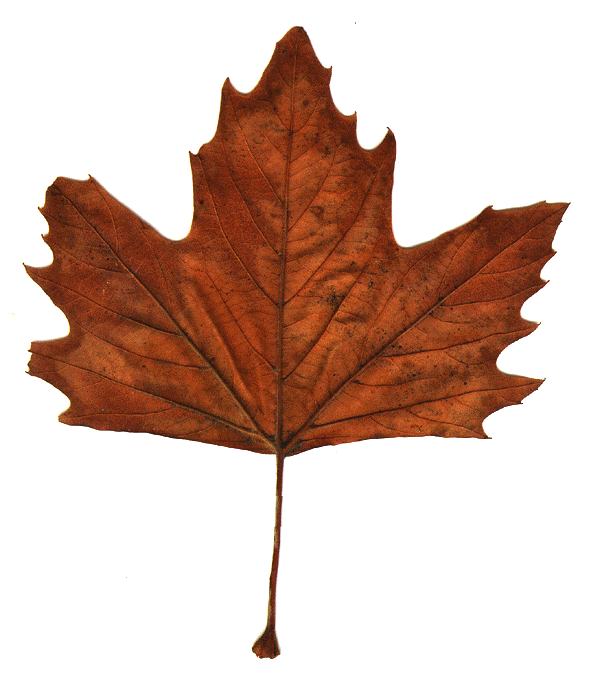Some other IFS examples of plant-like structures are shown below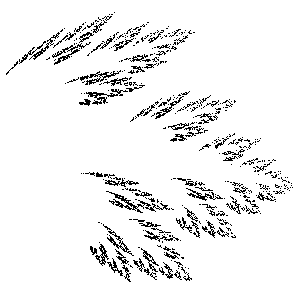References

Demko, S., Hodges, L., and Naylor B. Construction of Fractal Objects with Iterated Function Systems. Computer Graphics 19, 3, July 1985, Pages 271-278

Barnsley, M. Fractals Everywhere. Academic press, 1988

Barnsley, F., and Sloan, A.D. A Better Way to Compress Images. Byte Magazine, January 1988, Pages 215-222

Oppenheimer, P.E. Real Time Design and Animation of Fractal Plants and Trees. Computer Graphics, 20, 4, 1986

Reghbati, H.K. An Overview of Data Compression Techniques. Computer, 14, 4, 1981, Pages 71-76

# Gallery of Randomly Generated IFS

Created by Paul Bourke
March 1998

The following images are a selection from an infinite set of Random Iterated Function Systems (IFS) of the form

xn+1 = a xn + b yn + c

yn+1 = d xn + e yn + f

The image is formed by drawing a point at each iteration at the position (xi,yi).

For the mapping to contractive the following must all be true.

a2 + d2 < 1

b2 + e2 < 1

a2 + b2 + d2 + e2 < 1 + (ae - db)2

For any particular image there are 4 different sets of values for (a,b,c,d,e,f) all on the interval [-1,1]. At each iteration step one of the sets is chosen at random to form the next term in the sequence (series).

The results fall into the following broad classes of images

 Infinite or constant, these can't be drawn and are specifically tested for and ignored by the software. (These are not affine mappings) Single blobs Often with outgoing rays.Interesting, attractive, and often organic forms.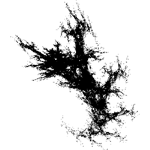The third "interesting" category can be further classified as follows

 Things made up of intersecting spikes They often look like Kanji text.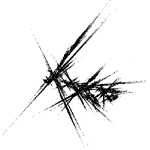Things that look like blobs of seaweed on the beach.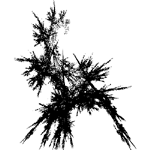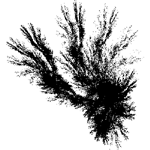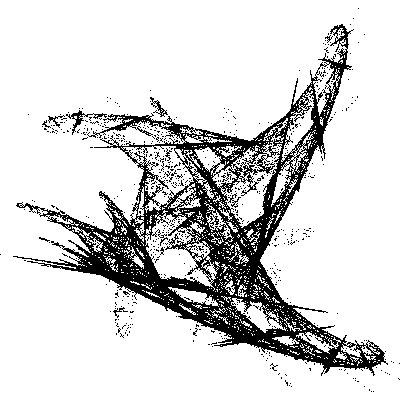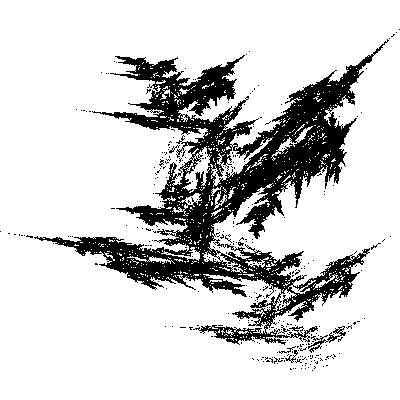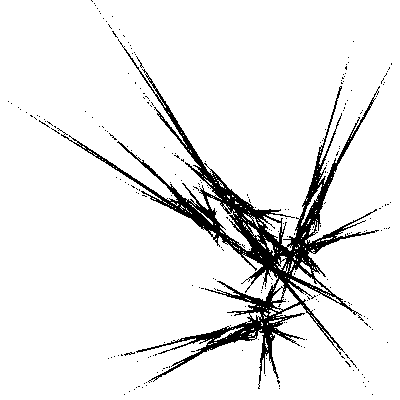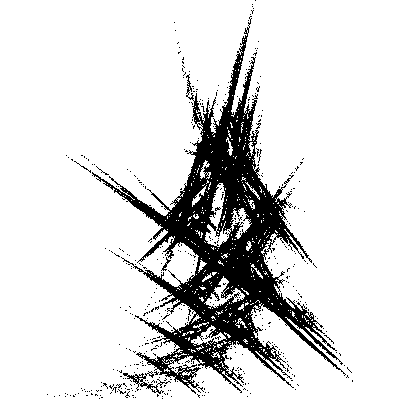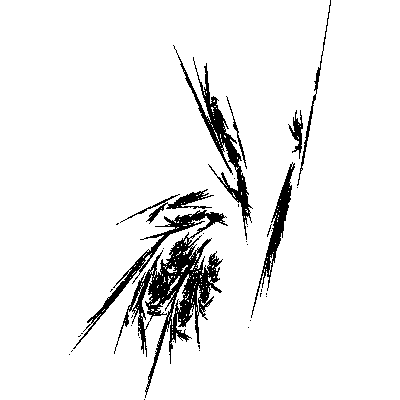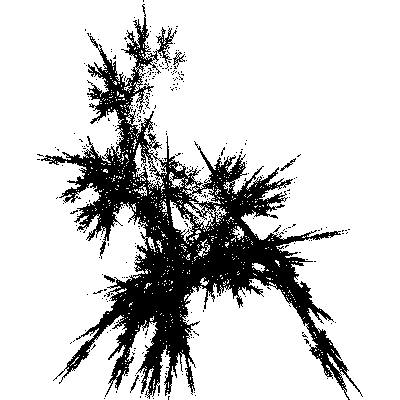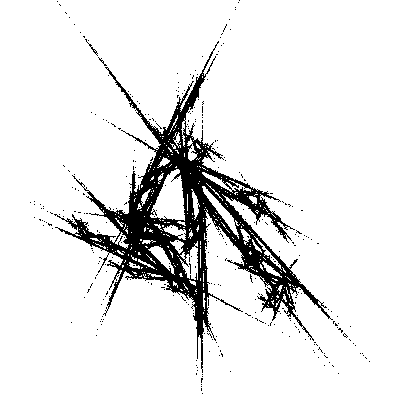# 3D IFS Bush (weed)

Written by Paul Bourke
May 2003
Original model from the authors of "IFS Builder 3d".This bush is created by iteratively choosing one of the following functions at random to form a series pn. Each point in the series is represented as a small sphere, the final collection is rendered using PovRay. In order minimise the number of spheres to be rendered (IFS tend to require a very large number of points) the spheres are filtered such that no two spheres lie within 10% of their respective radii.

• scale by (0.1,0.2,0.1)

• translate by (0,-1,0)
scale by (0.9,0.8,0.9)
rotate about the y axis by 144 degrees
translate by (0,1,0)

• rotate about the z axis by 60 degrees
scale by 0.3
translate by (0,0.2,0)

# General N tile IFS

Inspired by Roger Bagula
Compiled and graphics by Paul Bourke
Original October 1998, images updated January 2018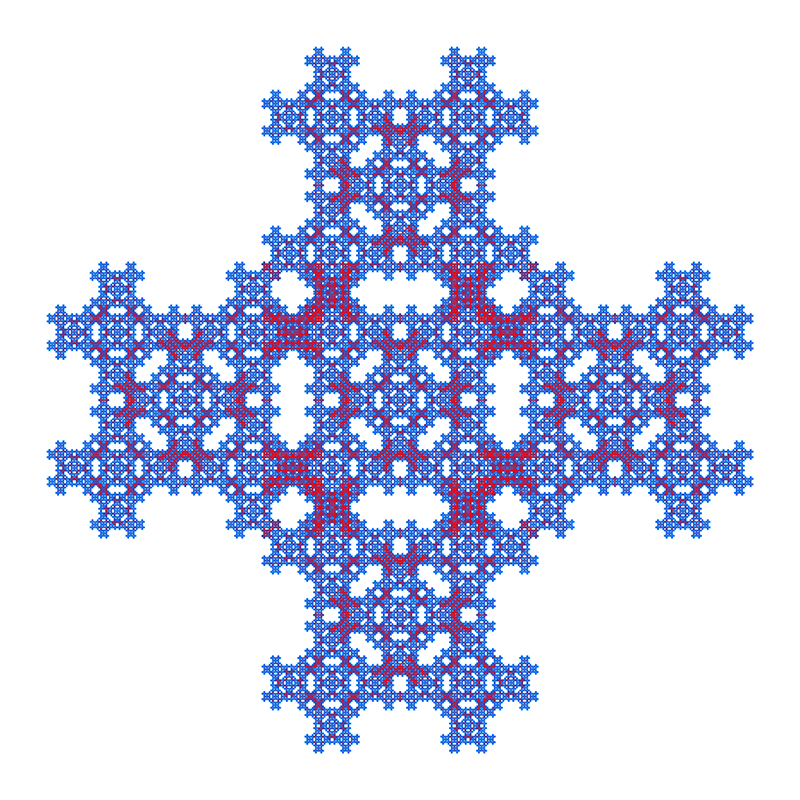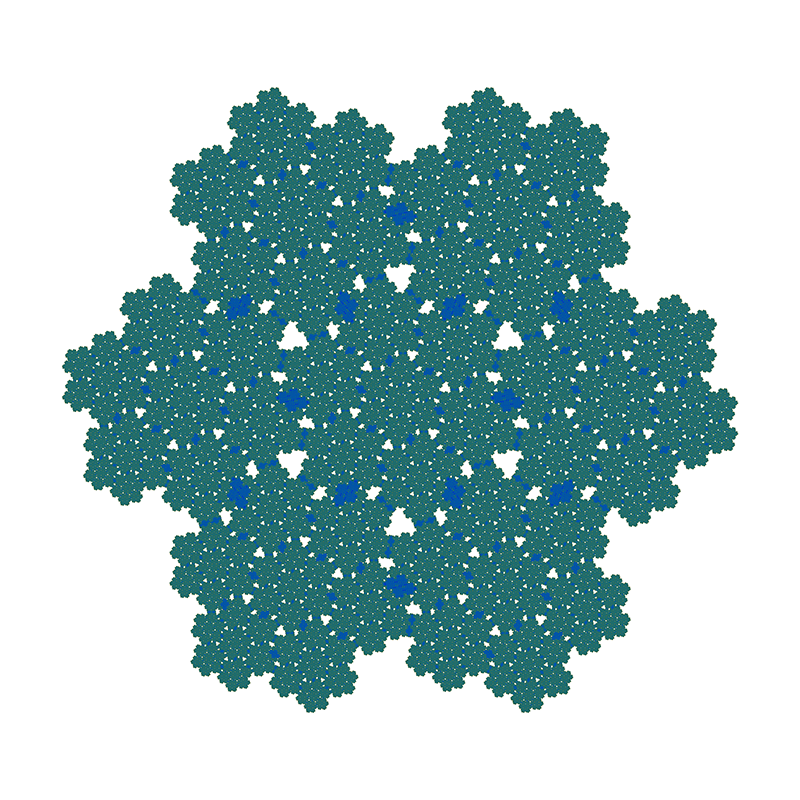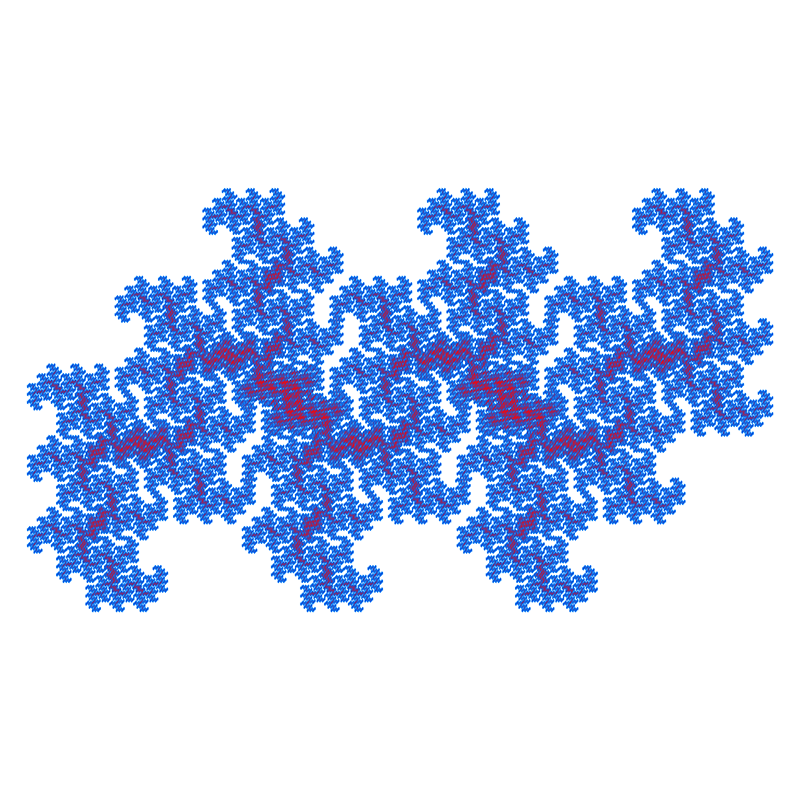# Maple Leaf IFS

Written by Paul Bourke
January 2002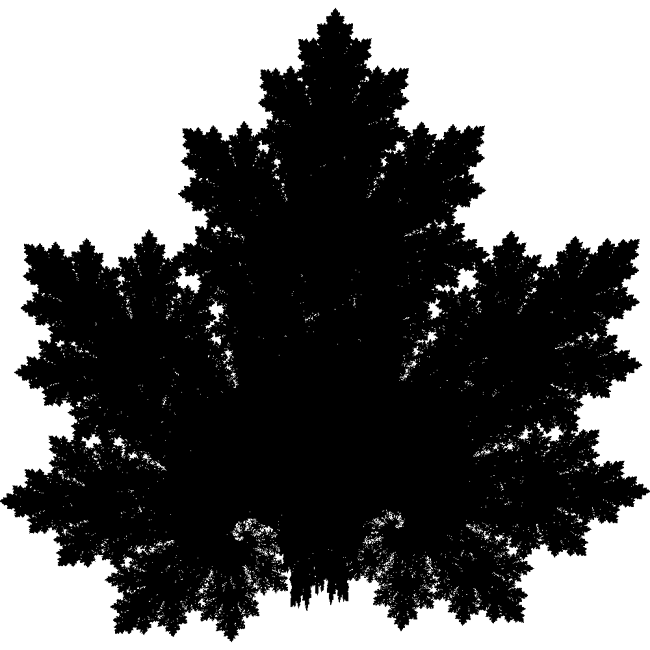The IFS equations are as follows

xn+1 = a xn + b yn + e

yn+1 = c xn + d yn + f

The parameter table:
```                   set 1     set 2     set 3     set 4
a     0.1400    0.4300    0.4500    0.4900
b     0.0100    0.5200   -0.4900    0.0000
c     0.0000   -0.4500    0.4700    0.0000
d     0.5100    0.5000    0.4700    0.5100
e    -0.0800    1.4900   -1.6200    0.0200
f    -1.3100   -0.7500   -0.7400    1.6200
```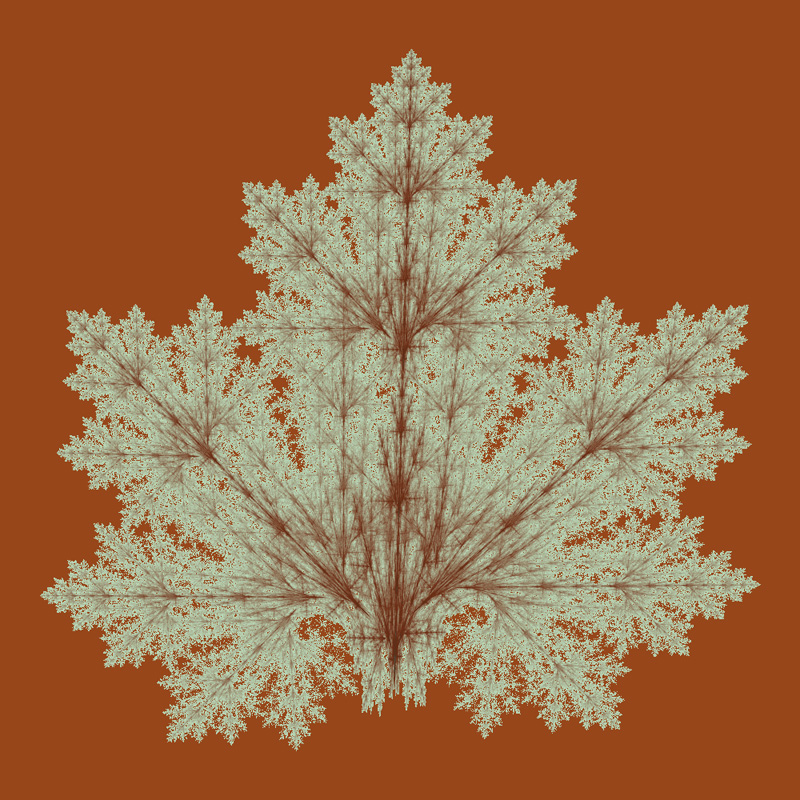Features in the Diaorama Magazine, issue 4, May 2013.

# IFS Spiral

Written by Paul Bourke
January 2002The IFS equations are as follows

xn+1 = a xn + b yn + e

yn+1 = c xn + d yn + f

The parameter table:
```                   set 1       set 2        set 3
a     0.787879   -0.121212     0.181818
b    -0.424242    0.257576    -0.136364
c     0.242424    0.151515     0.090909
d     0.859848    0.053030     0.181818
e     1.758647   -6.721654     6.086107
f     1.408065    1.377236     1.568035
probability     0.90        0.05         0.05
```

# IFS Mandelbrot-like

Written by Paul Bourke
February 1999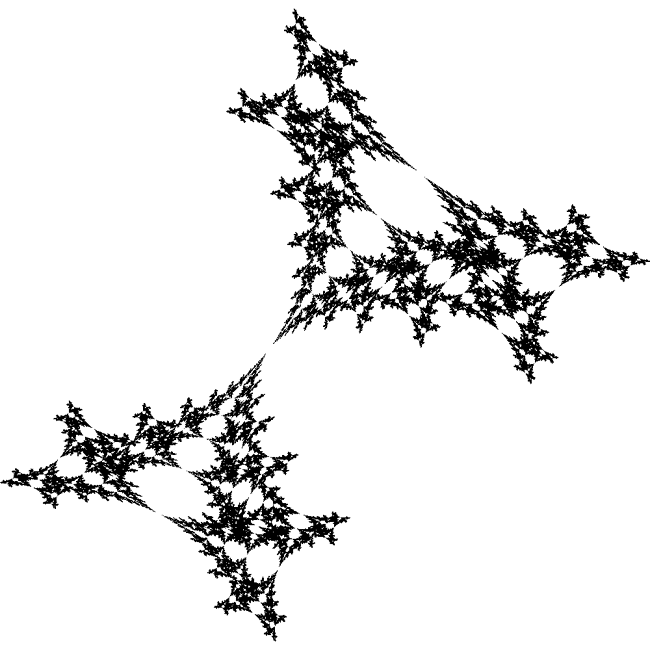The IFS equations are as follows

xn+1 = a xn + b yn + e

yn+1 = c xn + d yn + f

The parameter table:
```                   set 1     set 2
a     0.2020    0.1380
b    -0.8050    0.6650
c    -0.6890   -0.5020
d    -0.3420   -0.2220
e    -0.3730    0.6600
f    -0.6530   -0.2770
```

# IFS Tree

Written by Paul Bourke
January 1999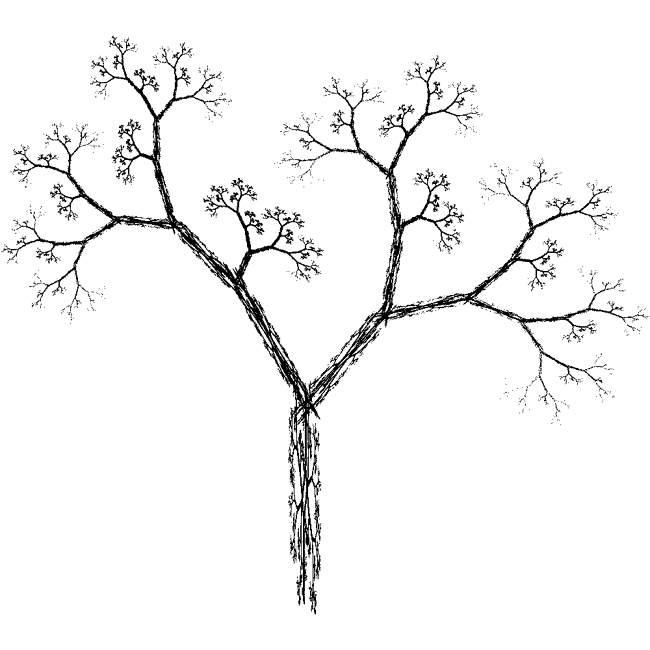The IFS equations are as follows

xn+1 = a xn + b yn + e

yn+1 = c xn + d yn + f

The parameter table:
```                   set 1     set 2     set 3     set 4     set 5     set 6     set 7
a     0.0500   -0.0500    0.0300   -0.0300    0.5600    0.1900   -0.3300
b     0.0000    0.0000   -0.1400    0.1400    0.4400    0.0700   -0.3400
c     0.0000    0.0000    0.0000    0.0000   -0.3700   -0.1000   -0.3300
d     0.4000   -0.4000    0.2600   -0.2600    0.5100    0.1500    0.3400
e    -0.0600   -0.0600   -0.1600   -0.1600    0.3000   -0.2000   -0.5400
f    -0.4700   -0.4700   -0.0100   -0.0100    0.1500    0.2800    0.3900
```Derby, Western Australia

# IFS Tree

Written by Paul Bourke
January 1999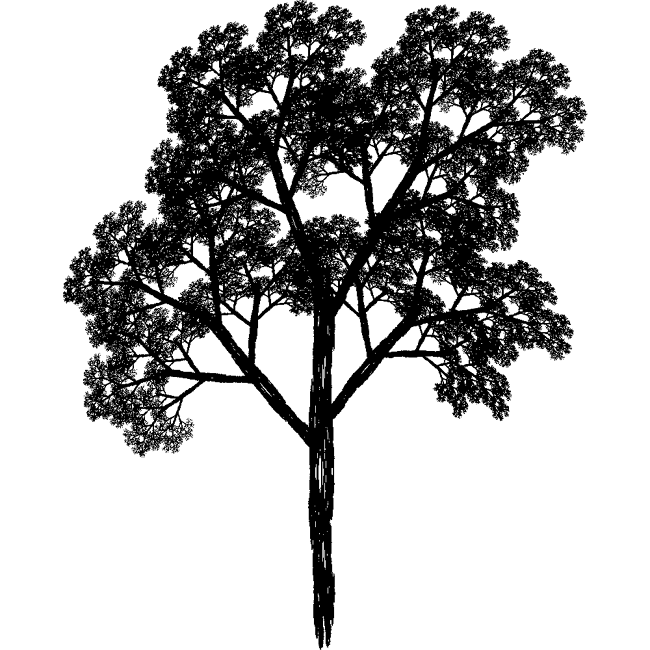The IFS equations are as follows

xn+1 = r cos(theta) xn - s sin(phi) yn + e

yn+1 = r sin(theta) xn + s cos(phi) yn + f

The parameter table:
```                   set 1     set 2     set 3     set 4     set 5     set 6
r     0.0500    0.0500    0.6000    0.5000    0.5000    0.5500
s     0.6000   -0.5000    0.5000    0.4500    0.5500    0.4000
theta     0.0000    0.0000    0.6980    0.3490   -0.5240   -0.6980
phi     0.0000    0.0000    0.6980    0.3492   -0.5240   -0.6980
e     0.0000    0.0000    0.0000    0.0000    0.0000    0.0000
f     0.0000    1.0000    0.6000    1.1000    1.0000    0.7000
```

# IFS Tree

Written by Paul Bourke
January 1999The IFS equations are as follows

xn+1 = a xn + b yn + e

yn+1 = c xn + d yn + f

The parameter table:
```                   set 1     set 2     set 3     set 4
a     0.0100   -0.0100    0.4200    0.4200
b     0.0000    0.0000   -0.4200    0.4200
c     0.0000    0.0000    0.4200   -0.4200
d     0.4500   -0.4500    0.4200    0.4200
e     0.0000    0.0000    0.0000    0.0000
f     0.0000    0.4000    0.4000    0.4000
```

# IFS Tree

Written by Paul Bourke
January 1999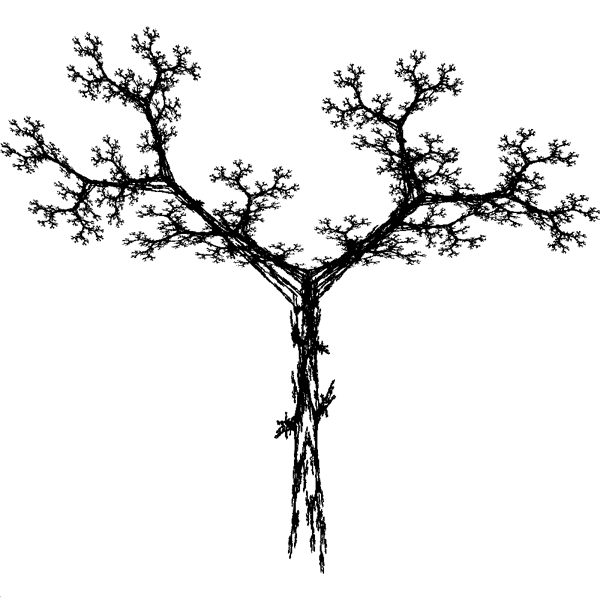The IFS equations are as follows

xn+1 = a xn + b yn + e

yn+1 = c xn + d yn + f

The parameter table:
```                   set 1     set 2     set 3     set 4     set 5
a     0.1950    0.4620   -0.6370   -0.0350   -0.0580
b    -0.4880    0.4140    0.0000    0.0700   -0.0700
c     0.3440   -0.2520    0.0000   -0.4690    0.4530
d     0.4430    0.3610    0.5010    0.0220   -0.1110
e     0.4431    0.2511    0.8562    0.4884    0.5976
f     0.2452    0.5692    0.2512    0.5069    0.0969
probability     0.2       0.2       0.2       0.2       0.2
```

# Leaf IFS

Written by Paul Bourke
January 2002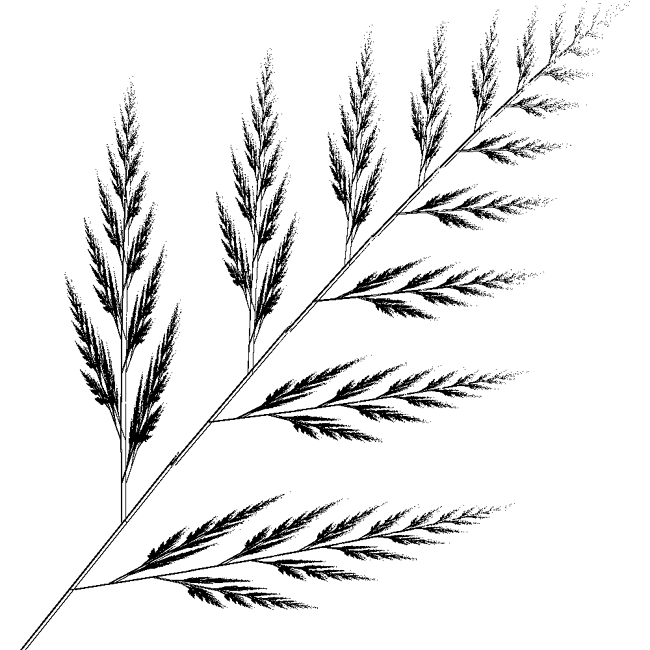The IFS equations are as follows

xn+1 = a xn + b yn + e

yn+1 = c xn + d yn + f

The parameter table:
```                   set 1       set 2      set 3      set 4
a     0.0000      0.7248     0.1583     0.3386
b     0.2439      0.0337    -0.1297     0.3694
c     0.0000     -0.0253     0.3550     0.2227
d     0.3053      0.7426     0.3676    -0.0756
e     0.0000      0.2060     0.1383     0.0679
f     0.0000      0.2538     0.1750     0.0826
```

# Sand dollar snowflake IFS

Written by Paul Bourke
January 2002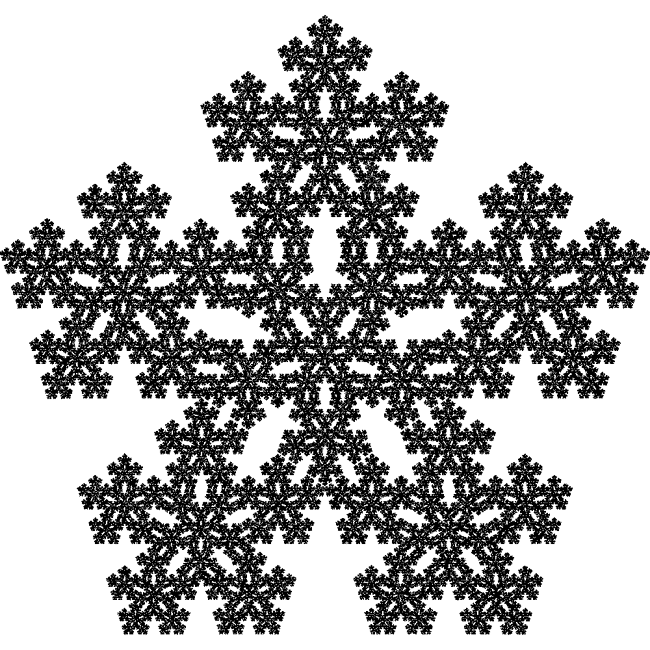The IFS equations are as follows

xn+1 = a xn + b yn + e

yn+1 = c xn + d yn + f

The parameter table:
```                   set 1       set 2      set 3      set 4      set 5      set 6
a     0.38200     0.11800    0.11800   -0.30900   -0.30900    0.38200
b     0.00000    -0.36330    0.36330   -0.22450    0.22450    0.00000
c     0.00000     0.36330   -0.36330    0.22450   -0.22450    0.00000
d     0.38200     0.11800    0.11800   -0.30900   -0.30900   -0.38200
e     0.30900     0.36330    0.51870    0.60700    0.70160    0.30900
f     0.57000     0.33060    0.69400    0.30900    0.53350    0.67700
```

# How to create the IFS Fern

Written by Paul Bourke
January 1988

Version by Jay Link designed for SVGALIB
and Object pascal version by Omar Awile.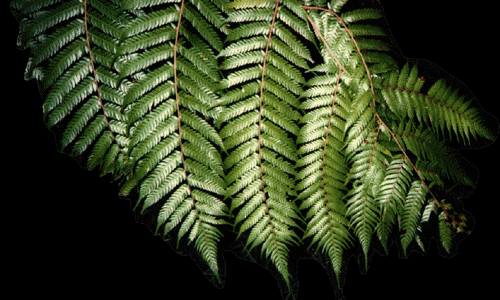One of the very common and attractive forms generated by Iterated Function Systems (IFS) is the fern leaf shown on the right. The following will describe how to generate this form and allow the reader to experiment with other IFS generators. Iterated function systems are described by repeatedly computing terms in two series, one series describes the x coordinate and the other series the y coordinate. The equations describe translation, scaling, rotation, and shearing of points in a plane with the restriction that the transformations are "affine". The general form of the series are as follows xn+1 = a xn + b yn + e yn+1 = c xn + d yn + f A point is drawn at each pair (xi,yi) for i greater than some number, typically 10 to 100. The magic is in finding the values of (a,b,c,d,e,f) that give the desired form. In many application it is necessary to have a number of sets of (a,b,c,d,e,f). As the series is being generated a particular set is chosen at random for each term. Such IFS systems are often known as Random Iterated Function Systems.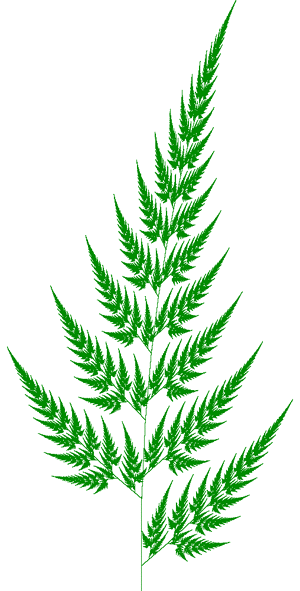The fern can be constructed using the table of value on the right.
It turns out that if the different sets of (a,b,c,d,e,f) are chosen with appropriate probabilities then the fern will "emerge" much faster and evenly than if the sets are chosen with equal chance. The last row in the table gives the optimal probabilities.

The fern above resulted from 100 thousand (105) iterations, that is, 100 thousand points are drawn (of course when drawn on the bitmap many will overlap)

 - set1 set2 set3 set4 a 0.0 0.2 -0.15 0.75 b 0.0 -0.26 0.28 0.04 c 0.0 0.23 0.26 -0.04 d 0.16 0.22 0.24 0.85 e 0.0 0.0 0.0 0.0 f 0.0 1.6 0.44 1.6 p 0.1 0.08 0.08 0.74

The image is self similar at all scales, one can zoom in as far as one wishes and the fronds will continue to resolve themselves. For example, the following image is a zoom in by 50. Note however that it takes an ever increasing number of iterations to resolve the image as the zoom factor increases, this image took 100 million (108) iterations.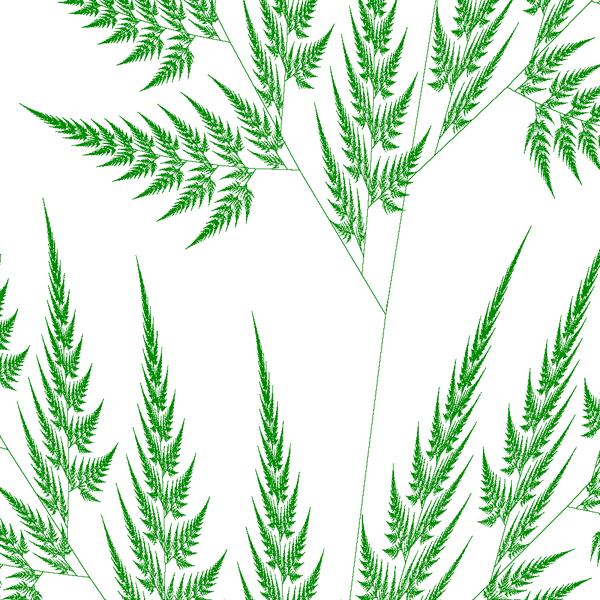Some straightforward C source is given here (source.c) which generates the figure shown above. Note, you will have to supply your own image drawing tools.

 A slightly different set of codes gives the result on the right. ``` set 1 set 2 set 3 set 4 a 0.0 0.2 -0.15 0.85 b 0.0 -0.26 0.28 0.04 c 0.0 0.23 0.26 -0.04 d 0.16 0.22 0.24 0.85 e 0.0 0.0 0.0 0.0 f 0.0 1.6 0.44 1.6 probability 0.01 0.07 0.07 0.85 ```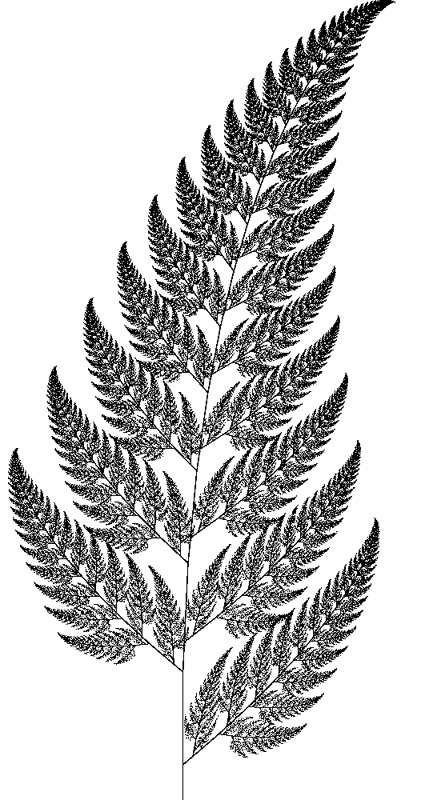Angular fern by Roger Bagula: Basic source code -- C source code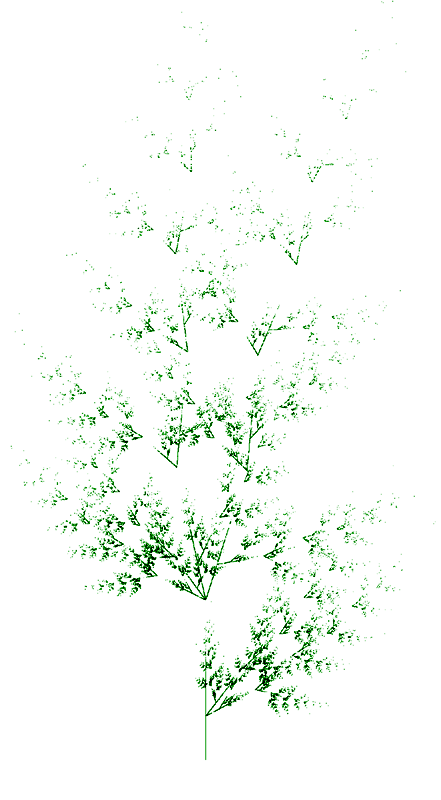References

Demko, S., Hodges, L., and Naylor B. Construction of Fractal Objects with Iterated Function Systems. Computer Graphics 19, 3, July 1985, Pages 271-278

Barnsley, M. Fractals Everywhere. Academic press, 1988

Barnsley, F., and Sloan, A.D. A Better Way to Compress Images. Byte Magazine, January 1988, Pages 215-222

>Oppenheimer, P.E. Real Time Design and Animation of Fractal Plants and Trees. Computer Graphics, 20, 4, 1986

Reghbati, H.K. An Overview of Data Compression Techniques. Computer, 14, 4, 1981, Pages 71-76

# IFS fern

Written by Paul Bourke
January 1999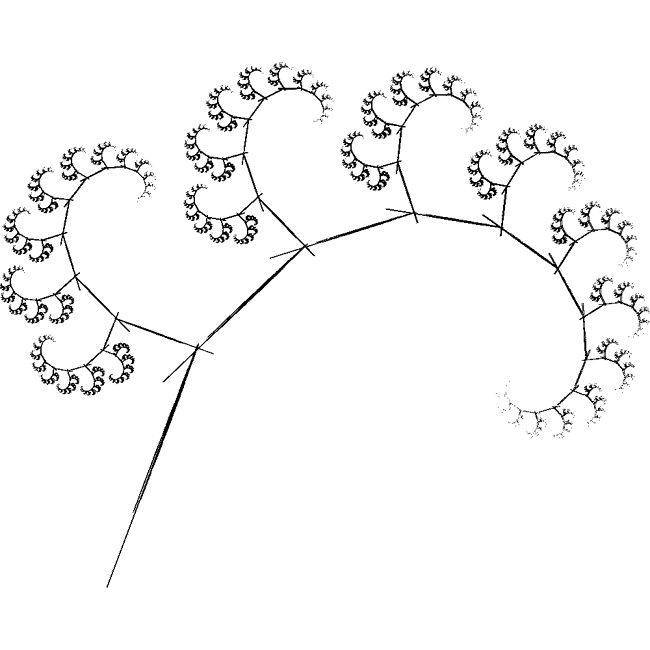The IFS equations are as follows

xn+1 = a xn + b yn + e

yn+1 = c xn + d yn + f

The parameter table:
```                   set 1     set 2     set 3
a     0.0100    0.7000    0.0000
b    -0.4100    0.3300    0.1750
c     0.3900   -0.3500    0.0130
d     0.0000    0.7000    0.4600
e    -0.2800    0.1850   -0.0950
f    -0.1850    0.0150   -0.2850
```

# IFS Chaos Text

Written by Paul Bourke
January 2002
Contributed by Earl Glynn (Original source unknown)

The following is a rather unusual example of an iterated function system (IFS) that involves choosing at random one of 19 transformations of the form

xn+1 = a xn + b yn + e
yn+1 = c xn + d yn + f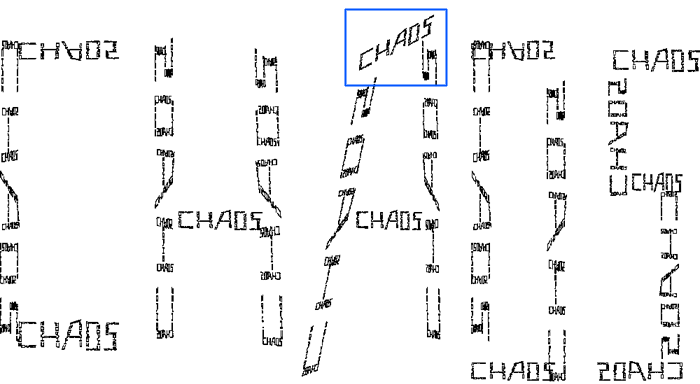A zoom into the top portion of the "A", shown in blue above, shows that it is indeed made up of smaller versions of the word "chaos".And to labour the point, the following is a further zoom into the "A", shown in blue above.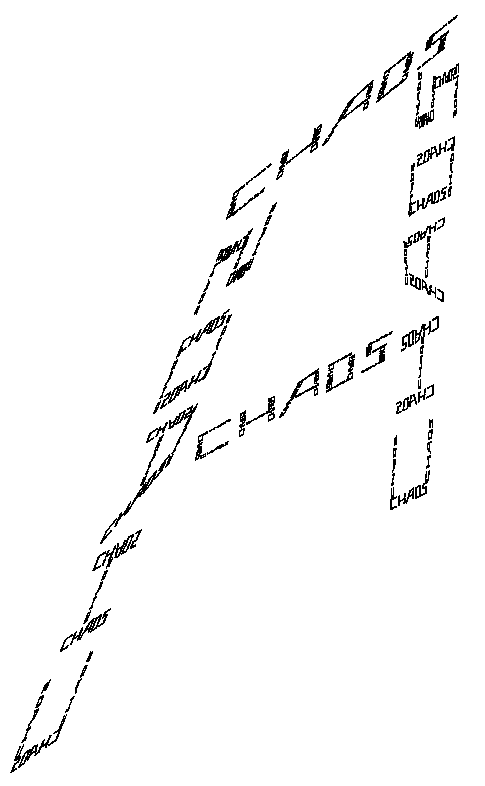The parameter table:

```    a           b           c            d            e         f
0           0.053      -0.429        0           -7.083     5.43
0.143       0           0           -0.053       -5.619     8.513
0.143       0           0            0.083       -5.619     2.057
0           0.053       0.429        0           -3.952     5.43
0.119       0           0            0.053       -2.555     4.536
-0.0123806  -0.0649723   0.423819     0.00189797  -1.226     5.235
0.0852291   0.0506328   0.420449     0.0156626   -0.421     4.569
0.104432    0.00529117  0.0570516    0.0527352    0.976     8.113
-0.00814186 -0.0417935   0.423922     0.00415972   1.934     5.37
0.093       0           0            0.053        0.861     4.536
0           0.053      -0.429        0            2.447     5.43
0.119       0           0           -0.053        3.363     8.513
0.119       0           0            0.053        3.363     1.487
0           0.053       0.429        0            3.972     4.569
0.123998   -0.00183957  0.000691208  0.0629731    6.275     7.716
0           0.053       0.167        0            5.215     6.483
0.071       0           0            0.053        6.279     5.298
0          -0.053      -0.238        0            6.805     3.714
-0.121       0           0            0.053        5.941     1.487
```

# IFS Dragon

Written by Paul Bourke
January 2002The IFS equations are as follows

xn+1 = a xn + b yn + e

yn+1 = c xn + d yn + f

The parameter table:
```                   set 1       set 2
a     0.824074    0.088272
b     0.281428    0.520988
c    -0.212346   -0.463889
d     0.864198   -0.377778
e    -1.882290    0.785360
f    -0.110607    8.095795
probability     0.8         0.2
```# IFS Twig

Written by Paul Bourke
January 2002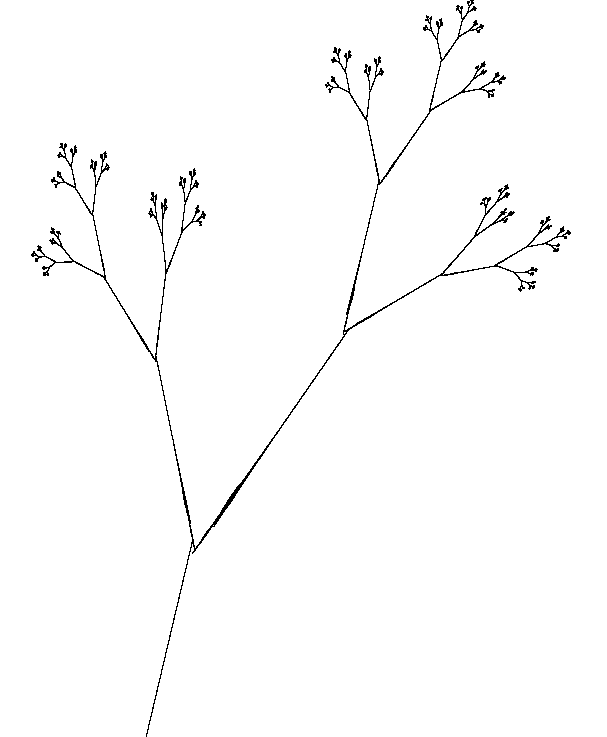The IFS equations are as follows

xn+1 = a xn + b yn + e

yn+1 = c xn + d yn + f

The parameter table:
```                   set 1       set 2      set 3
a     0.387       0.441     -0.468
b     0.430      -0.091      0.020
c     0.430      -0.009     -0.113
d    -0.387      -0.322      0.015
e     0.2560      0.4219     0.4
f     0.5220      0.5059     0.4
probability     1/3         1/3        1/3
```

# Christmas tree IFS

Written by Paul Bourke
January 2002The IFS equations are as follows

xn+1 = a xn + b yn + e

yn+1 = c xn + d yn + f

The parameter table:
```                   set 1       set 2      set 3
a     0.0         0.0        0.5
b    -0.5         0.5        0.0
c     0.5        -0.5        0.0
d     0.0         0.0        0.5
e     0.5         0.5        0.25
f     0.0         0.5        0.5
probability     1/3         1/3        1/3
```

# 3D IFS Hedgehog

Written by Paul Bourke
May 2003

Name attributed to the authors of "IFS Builder 3d".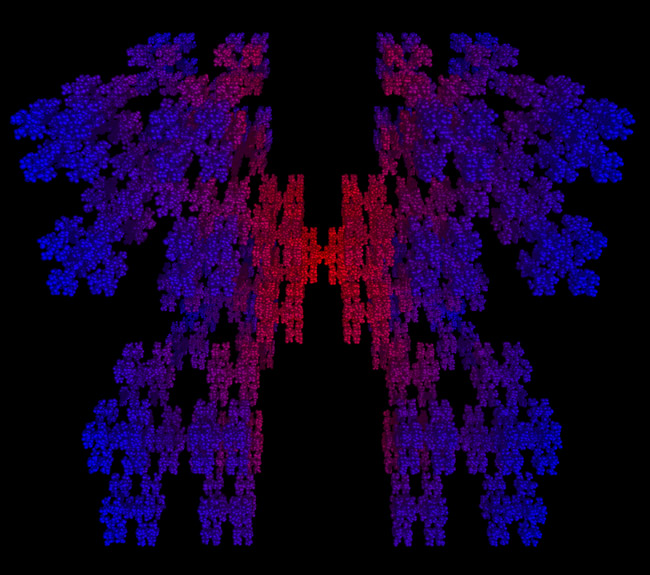This beast is created by iteratively choosing one of the following functions at random to form a series pn. Each point in the series is represented as a small sphere, the final collection is rendered using PovRay. In order minimise the number of spheres to be rendered (IFS tend to require a very large number of points) the spheres are filtered such that no two spheres lie within 10% of their respective radii.

• scale by 1/3
• translate by (a,a,a)
scale by b
• translate by (a,a,-a)
scale by b
• translate by (a,-a,a)
scale by b
• translate by (a,-a,-a)
scale by b
• translate by (-a,a,a)
scale by b
• translate by (-a,a,-a)
scale by b
• translate by (-a,-a,a)
scale by b
• translate by (-a,-a,-a)
scale by b

where a = (1 + 1/3) / 2 and b = (1 - 1/3) / 2

# 3D IFS Cross

Written by Paul Bourke
May 2003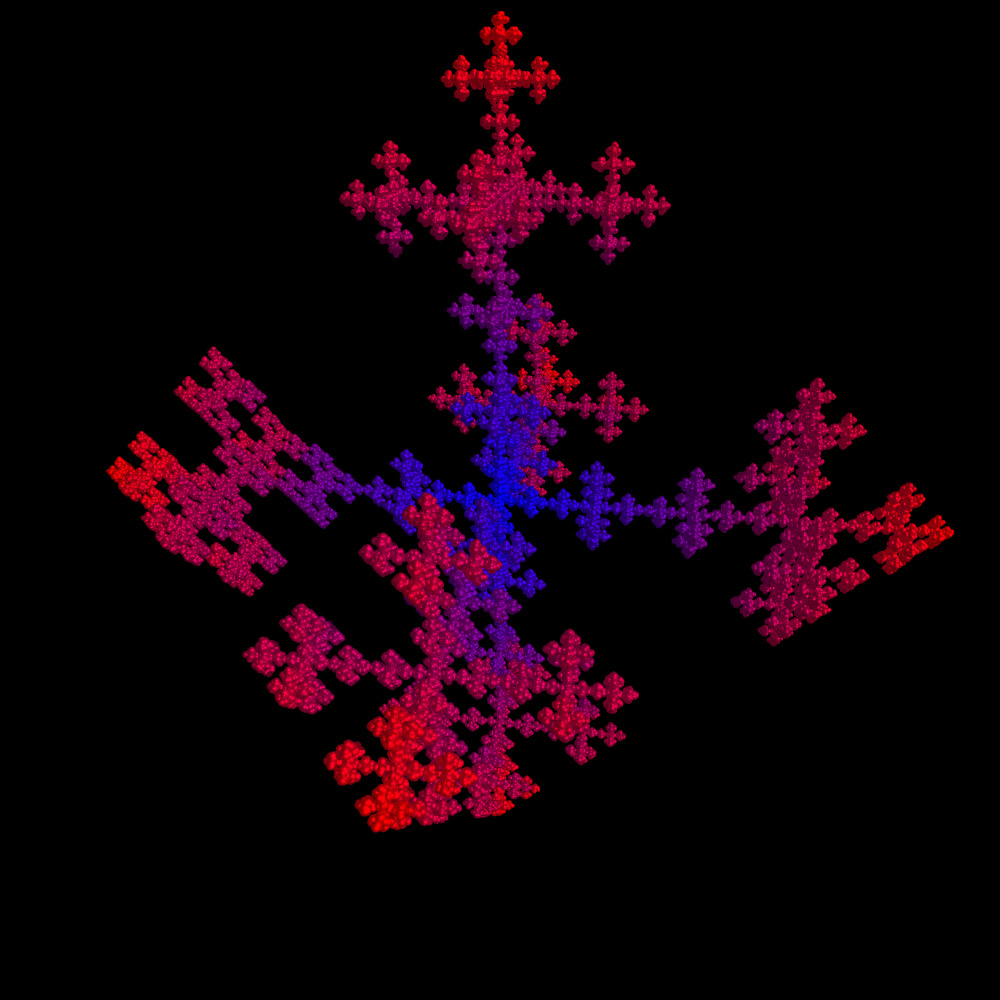This beast is created by iteratively choosing one of the following functions at random to form a series pn. Each point in the series is represented as a small sphere, the final collection is rendered using PovRay. In order minimise the number of spheres to be rendered (IFS tend to require a very large number of points) the spheres are filtered such that no two spheres lie within 10% of their respective radii.

• scale by 1/3
• translate by (a,0,0)
scale by b
• translate by (0,a,0)
scale by b
• translate by (0,0,a)
scale by b
• translate by (-a,0,0)
scale by b
• translate by (0,-a,0)
scale by b
• translate by (0,0,-a)
scale by b

where a = (1 + 1/3) / 2 and b = (1 - 1/3) / 2